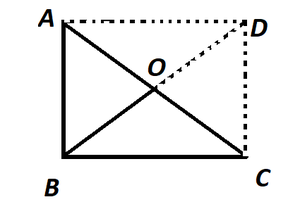Open in App
Not now

# Class 8 NCERT Solutions – Chapter 3 Understanding Quadrilaterals – Exercise 3.4

• Last Updated : 22 Dec, 2022

Question 1. State whether True or False.

(a) All rectangles are squares.

Answer: False, In square all sides are equal, but in rectangle length and breadth are different.

(b) All rhombuses are parallelograms

Answer: True, A parallelogram has opposite sides parallel and equal in length. Also, the opposite angles are equal.

(c) All squares are rhombuses and also rectangles

Answer: True, Squares have a property similar to Rhombuses and also to rectangles.

(d) All squares are not parallelograms.

Answer: False, Squares have similar property as Parallelogram.

(e) All kites are rhombuses

Answer: False, All sides are not equal in Kite.

(f) All rhombuses are kites.

Answer: True, In the rhombus, diagonals bisect each other which is similar to kites.

(g) All parallelograms are trapeziums.

Answer: True, Trapezium has two parallel sides similar to parallelogram.

(h) All squares are trapeziums.

Answer: True, All squares have two sides which are parallel to each similar to Trapezium.

### Question 2. Identity all the quadrilaterals that have

(a) four sides of equal length

Answer: Rhombus and square have all sides equal.

(b)four right angles

Answer: Square and rectangle have four right angles.

### Question 3. Explain how a square is.

Answer: A square is a quadrilateral, as it has four equal sides.

(ii) a parallelogram

Answer: A square is a parallelogram, as it contains the pairs of opposite sides equal.

(iii) a rhombus

Answer: A square is a rhombus, as it has four equal sides and diagonals bisect at 900.

(iv) a rectangle

Answer: A square is a rectangle, as it has each adjacent angle at 900 and opposite sides are equal.

### Question 4. Name the quadrilaterals whose diagonals.

(i) bisect each other

Answer: It is a rhombus, parallelogram, rectangle, or square as their diagonals bisect each other.

(ii) are perpendicular bisectors of each other

Answer: It is a rhombus or square their diagonals are perpendicular bisector of each other

(iii) are equal

Answer: It is a square or rectangle because they have equal diagonals.

### Question 5 Explain why a rectangle is a convex quadrilateral.

Answer: A rectangle is a convex quadrilateral, as its vertex is raised and both of its diagonals lie in its interior.

### Question 6. ABC is a right-angled triangle and O is the midpoint of the side opposite to the right angle. Explain why O is equidistant from A, B, and C. (The dotted lines are drawn additionally to help you).Solution:

Draw two lines AD and DC such that AD II BC and AB II DC

AD = BC , AB = DC

Since, ABCD is a rectangle. Opposite sides are parallel and equal to each other and all the interior angles are right angles.

It is the property of rectangle that the diagonals are of equal length and bisect each other.

Hence, AO = OC = BO = OD.

Thus, O is equidistant from A, B and C.

My Personal Notes arrow_drop_up